## GPU (Cuda) Configuration for Deep Learning Frameworks (Tensorflow, Pytorch)

Speed your algorithms with GPU. Using GPU increases your computation speed x45-x50 times more than using CPU.

Look at the speed benchmark: Deep Learning Tensorflow Benchmark: Intel i5 4210U Vs GeForce Nvidia 1060 6GB. Sometimes, enabling GPU Cuda platform can be difficult.

Configuration List:

1. Download Microsoft Visual Studio with SDK (Visual Studio 2017 Recommended)
1. Once the IDE is installed successfully, the components for C++ development and Windows 10 SDK (Version 10.0.15063.0) must be installed.
2. Download NVIDIA GeForce Experience
3. Download CUDA Toolkit 10.0
4. Add to environment variables>path:
1. C:\Program Files\NVIDIA GPU Computing Toolkit\CUDA\v10.0\bin
2. C:\Program Files\NVIDIA GPU Computing Toolkit\CUDA\v10.0\lib\x64
3. C:\Program Files\NVIDIA GPU Computing Toolkit\CUDA\v10.0\include
5. Download NVIDIA cuDNN
6. Copy files from NVIDIA cuDNN
1. Copy <installpath>\cuda\bin\cudnn64_7.dll to C:\Program Files\NVIDIA GPU Computing Toolkit\CUDA\v10.0\bin.
2. Copy <installpath>\cuda\ include\cudnn.h to C:\Program Files\NVIDIA GPU Computing Toolkit\CUDA\v10.0\include
3. Copy <installpath>\cuda\lib\x64\cudnn.lib to C:\Program Files\NVIDIA GPU Computing Toolkit\CUDA\v10.0\lib\x64.
7.  Download and update Anaconda:
1. conda update conda
8.  Download Pytorch or Tensorflow.
1. pip install tensorflow-gpu
9. Pytorch CUDA control:
1. torch.cuda.is_available() returns True if GPU CUDA is configured correctly.
10. Tensorflow CUDA control:
1. tf.test.gpu_device_name() returns /device:GPU:0

Reference:

https://towardsdatascience.com/python-environment-setup-for-deep-learning-on-windows-10-c373786e36d1

## Reinforcement Learning (RL) Tutorial

Machine learning mainly consists of three methods: Supervised Learning, Unsupervised Learning and Reinforcement Learning. Supervised Learning provides mapping functionality between input and output using labelled dataset. Some of the supervised learning methods: Linear Regression, Support Vector Machines, Neural Networks, etc. Unsupervised Learning provides grouping and clustering functionality. Some of the supervised learning methods: K-Means, DBScan, etc. Reinforcement Learning is different from supervised and unsupervised learning. RL provides behaviour learning.

Keywords: Dynamic Programming (Policy and Value Iteration), Monte Carlo, Temporal Difference (SARSA, QLearning), Approximation, Policy Gradient, DQN.

NOTE: There are many tutorials in the internet. This one summarizes all of the tutorials with demo of RL algorithms.

## What is RL?

“A reinforcement learning algorithm, or agent, learns by interacting with its environment. The agent receives rewards by performing correctly and penalties for performing incorrectly. The agent learns without intervention from a human by maximizing its reward and minimizing its penalty” *. RL agents are used in different applications: Robotics, self driving cars, playing atari games, managing investment portfolio, control problems. I am believing that like many AI laboratories do, reinforcement learning with deep learning will be a core technology in the future.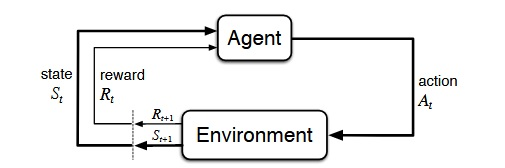[Sutton & Barto Book: RL: An Introduction]

### Markov Decision Process:

• It consists of five tuples: status, actions, rewards, state transition probability, discount factor.
• Markov decision processes formally describe an environment for reinforcement learning.
• There are 3 techniques for solving MDPs: Dynamic Programming (DP) Learning, Monte Carlo (MC) Learning, Temporal Difference (TD) Learning.

#### Markov Property:

A state St is Markov if and only if P[St+1|St] =P[St+1|S1,...,St]

## RL Components:

### Rewards:

• A reward Rt is a scalar feedback signal.
• The agent’s job is to maximise cumulative reward

### State Transition Probability:

• p(s′,r|s,a).= Pr{St=s′,Rt=r|St-1=s,At−1=a},

### Discount Factor:

• The discount γ∈[0,1] is the present value of future rewards.

### Return:

• The return Gt is the total discounted reward from time-step t.

### Value Function:

• Value function is a prediction of future reward. How good is each state and/or action.
• The value function v(s) gives the long-term value of state s
• Vπ(s) =Eπ[Rt+1+γRt+2+γ2Rt+3+...|St=s]
• Value function has two parts: immediate reward and discounted value of successor state.

### Policy (π):

A policy is the agent’s behaviour. It is a map from state to action.

• Deterministic policy: a=π(s).
• Stochastic policy: π(a|s) =P[At=a|St=s].

### Planning vs RL:

#### Planning:

• Rules of the game are known.
• A model of the environment is known.
• The agent performs computations with its mode.
• The agent improves its policy.

#### RL:

• The environment is initially unknown.
• The agent interacts with the environment.
• The agent improves its policy.

### Exploration and Exploitation:

• Reinforcement learning is like trial-and-error learning.
• The agent should discover a good policy.
• Exploration finds more information about the environment (Gather more information).
• Exploitation exploits known information to maximise reward (Make the best decision given current information).

### Prediction & Control Problem (Pattern of RL algorithms):

• Prediction: evaluate the future (Finding value given a policy).
• Control: optimise the future (Finding optimal/best policy).

## Grid World:

• Grid World is a game for demonstration. 12 positions, 11 states, 4 actions. Our aim is to find optimal policy.
• Demo Code: gridWorldGame.py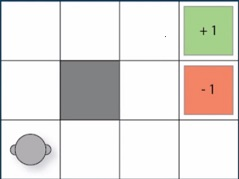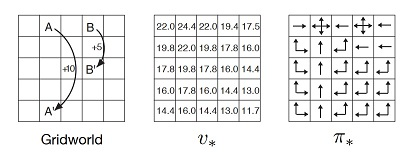## Dynamic Programming Method (DP): Full Model

• Dynamic Programming is a very general solution method for problems which have two properties: 1.Optimal substructure, 2.Overlapping subproblems.
• Markov decision processes satisfy both properties. Bellman equation gives recursive decomposition. Value function stores and reuses solutions.
• In DP method, full model is known, It is used for planning in an MDP.
• There are 2 methods: Policy Iteration, Value Iteration.
• DP uses full-width backups.
• DP is effective for medium-sized problems (millions of states).
• For large problems DP suffers Bellman’s curse of dimensionality.
• Disadvantage of DP: requires full model of environment, never learns from experience.

### Policy Iteration (with Pseudocode):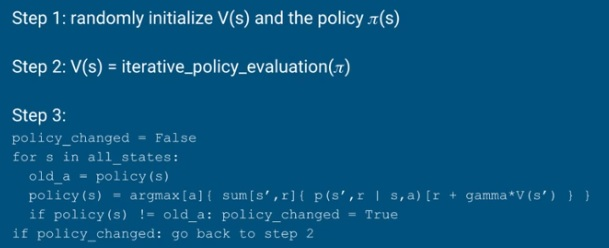#### Policy Evaluation (with Pseudocode):

• Problem: evaluate a given policy π.
• Solution: iterative application of Bellman expectation backup.
• v1 → v2→ … → vπ.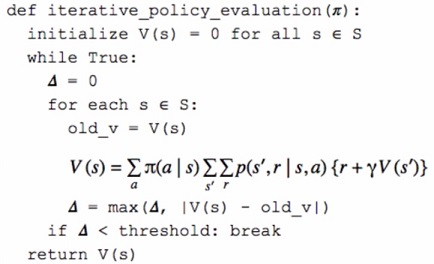#### Policy Improvement: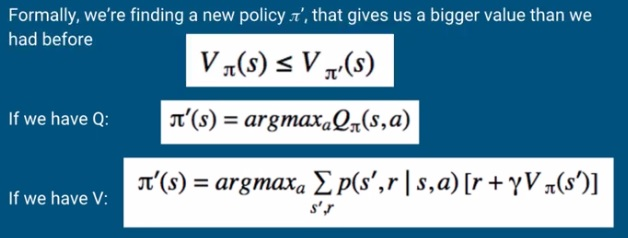### Value Iteration (with Pseudocode):

• Policy iteration has 2 inner loop. However, value iteration has a better solution.
• It combines policy evaluation and policy improvement into one step.
• Problem: find optimal policy π.
• Solution: iterative application of Bellman optimality backup.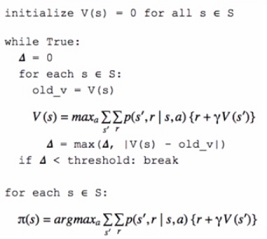## Monte Carlo (MC) Method:

• Demo Code: monte_carlo_demo.ipynb
• MC methods learn directly from episodes of experience.
• MC is model-free : no knowledge of MDP transitions / rewards.
• MC uses the simplest possible idea: value = mean return.
• Episode must terminate before calculating return.
• Average return is calculated instead of using true return G.
• First Visit MC: The first time-step t that state s is visited in an episode.
• Every Visit MC: Every time-step t that state s is visited in an episode.

### MC Calculating Returns (with Pseudocode):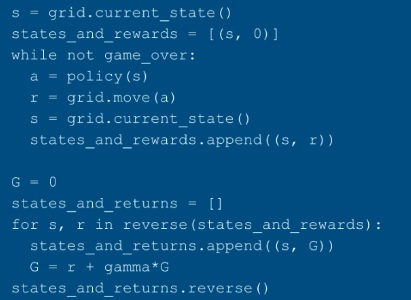### First-Visit MC (with Pseudocode) (MC Prediction Problem):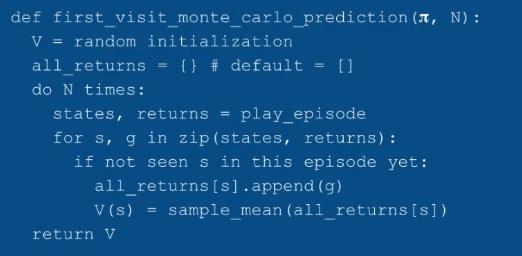### Exploring-Starts (with Pseudocode) (MC Control Problem):

• Demo Code: monte_carlo_es_demo.ipynb
• State s and Action a is randomly selected for all starting points.
• Use Q instead of V
• Update the policy after every episode, keep updating the same Q in-place.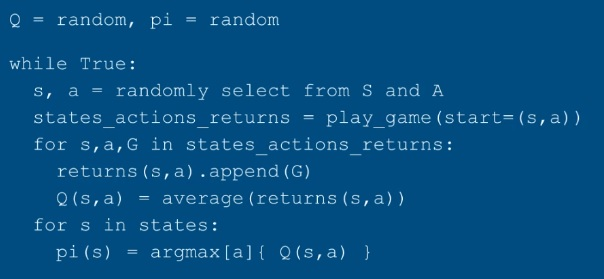### MC Epsilon Greedy (without Exploring Starts):

• Demo Code: monte_carlo_epsilon_greedy_demo.ipynb
• MC Exploring start is infeasible, because in real problems we can not calculate all edge cases (ex: in self-driving car problem, we can not calculate all cases).
• Randomly selection for all starting points in code is removed.
• Change policy to sometimes be random.
• This random policy is Epsilon-Greedy (like multi-armed bandit problem)

## Temporal Difference (TD) Learning Method:

• Demo Code: td0_prediction.ipynb
• TD methods learn directly from episodes of experience.
• TD updates a guess towards a guess
• TD learns from incomplete episodes, by bootstrapping.
• TD uses bootstrapping like DP, TD learns experience like MC (combines MC and DP).

## MC – TD Difference:

• MC and TD learn from experience.
• TD can learn before knowing the final outcome.
• TD can learn online after every step. MC must wait until end of episode before return is known.
• TD can learn without the final outcome.
• TD can learn from incomplete sequences. MC can only learn from complete sequences.
• TD works in continuing environments. MC only works for episodic environments.
• MC has high variance, zero bias. TD has low variance, some bias.

## MC – TD – DP Difference in Visual:

### SARSA (TD Control Problem, On-Policy):

• Demo Code: SARSA_demo.ipynb
• In on-policy learning the Q(s,a) function is learned from actions, we took using our current policy π.

### Q-Learning (TD Control Problem, Off-Policy):

• Demo Code: q_learning_demo.ipynb
• Looks like SARSA, instead of choosing a’ based on argmax of Q, Q(s,a) is updated directly with max over Q(s’,a’)
• In off-policy learning the Q(s,a) function is learned from different actions (for example, random actions). We even don’t need a policy at all.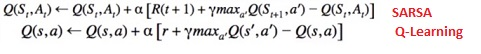## Function Approximation:

• Demo Code: func_approx_q_learning_demo.ipynb
• Reinforcement learning can be used to solve large problems, e.g Backgammon: 1020 states; Computer Go: 10170 states; Helicopter: continuous state space.
• So far we have represented value function by a lookup table, Every state s has an entry V(s) or every state-action pair s, a has an entry Q(s,a).
• There are too many states and/or actions to store in memory. It is too slow to learn the value of each state individually. Tabulated Q may not fit memory.
• Solution for large MDPs: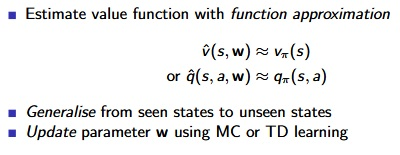• Differentiable function approximators can be used: Linear combinations of features, Neural Networks.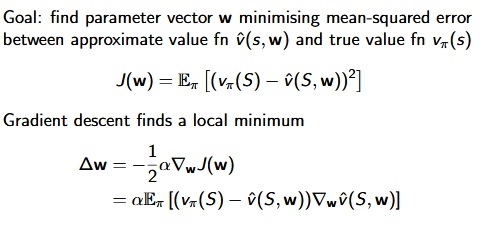## Open AI Gym Environment: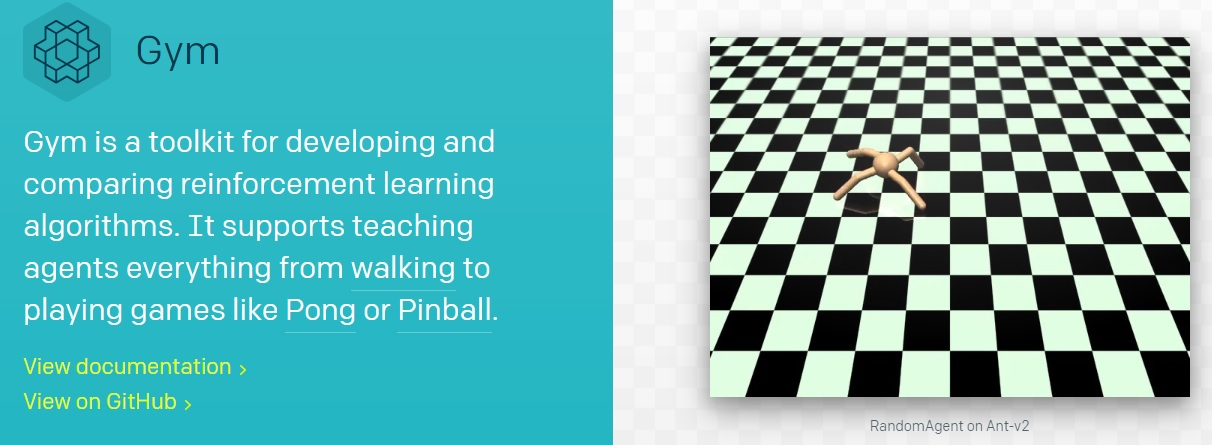## Policy Gradient:

• DP, MC and TD Learning methods are value-based methods (Learnt Value Function, Implicit policy).
• In value-based methods, a policy was generated directly from the value function (e.g. using epsilon-greedy)
• In policy-based, we will directly parametrise the policy ( πθ(s,a) =P[a|s,θ) ).
• Policy Gradient method is a policy-based method (No Value Function, Learnt Policy).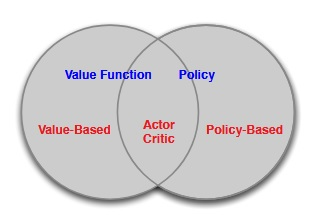Advantages:

• Better convergence properties,
• Effective in high-dimensional or continuous action spaces,
• Can learn stochastic policies.

Disadvantages:

• Typically converge to a local rather than global optimum.
• Evaluating a policy is typically inefficient and high variance.

### Policy Objective Functions:

• Policy based reinforcement learning is an optimisation problem.
• Find θ that maximises J(θ).

### Policy-Gradient: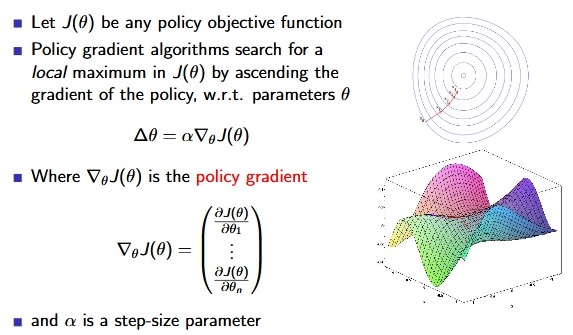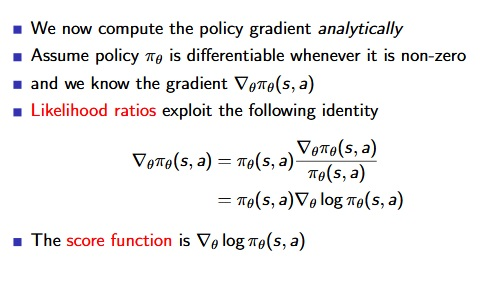## Actor-Critic:

• Actor-Critic method is a policy-based method (Learnt Value Function, Learnt Policy).
• The critic is solving a familiar problem: policy evaluation.

### Action-Value Actor-Critic: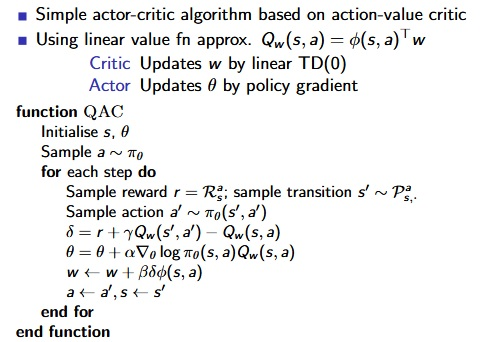• The advantage function can significantly reduce variance of policy gradient.
• So the critic should really estimate the advantage function.

### Actor-critic algorithm: A3C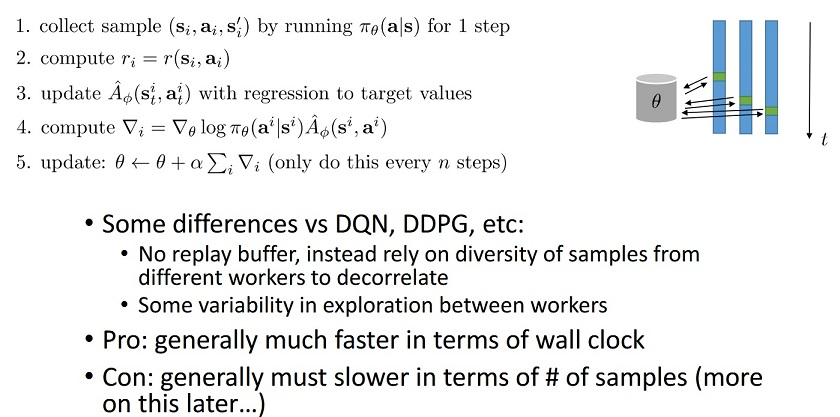## Model-Based RL: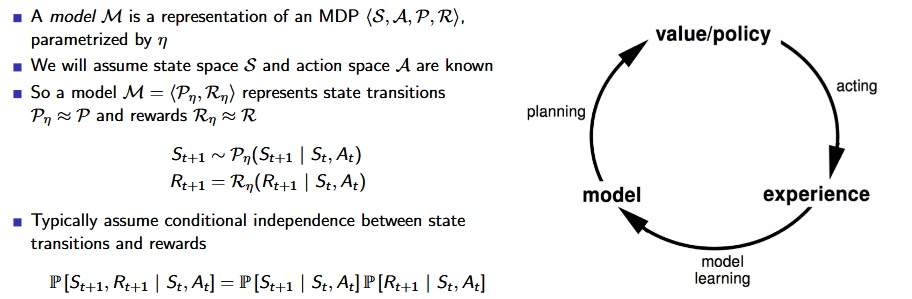• Favourite planning algorithms: Value iteration,Policy iteration,Tree search,etc..
• Sample-based Modeling: A simple but powerful approach to planning. Use the model only to generate samples. Sample experience from model.
• Apply model-free RL to samples, e.g.: Monte-Carlo control, SARSA, Q-Learning.
• Model-based RL is only as good as the estimated model.
• When the model is inaccurate, planning process will compute a suboptimal policy: 1.when model is wrong, use model-free RL; 2.reason explicitly about model uncertainty.

### Real and Simulated Experience: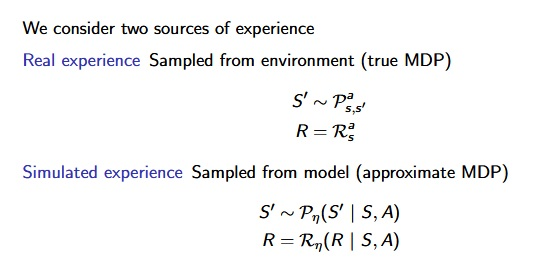### MC-Tree-Search:

• AlphaGo- Supervised learning + policy gradients + value functions + Monte Carlo tree search D. Silver, A. Huang, C. J.Maddison, A. Guez, L. Sifre, et al. “Mastering the game of Go with deep neural networks and tree search”. Nature (2016).
• Highly selective best-first search.
• Evaluates states dynamically (unlike e.g. DP).
• Uses sampling to break curse of dimensionality.
• Computationally efficient, anytime, parallelisable.
• Works for “black-box” models (only requires samples).

### Temporal-Difference Search:

• Simulation-based search.
• Using TD instead of MC (bootstrapping).
• MC tree search applies MC control to sub-MDP from now.
• TD search applies Sarsa to sub-MDP from now.
• For simulation-based search, bootstrapping is also helpful.
• TD search is usually more efficient than MC search.
• TD(λ) search can be much more efficient than MC search.

## Deep Q Learning (Deep Q-Networks: DQN):

• Gradient descent is simple and appealing. But it is not sample efficient.
• Batch methods seek to find the best fitting value function.
• Given the agent’s experience (“training data”)

### Experience Replay: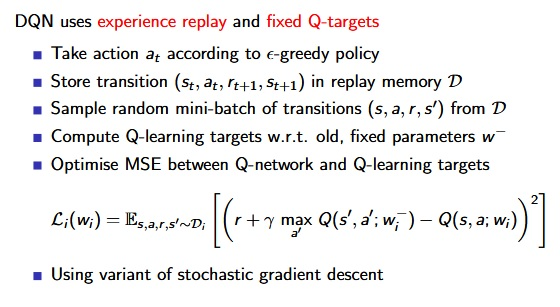### DQN in Atari:

-V. Mnih, K. Kavukcuoglu, D. Silver, A. Graves, I. Antonoglou, et al. “Playing Atari with Deep Reinforcement Learning”. (2013)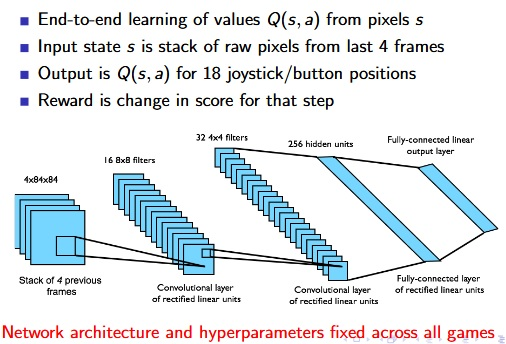## Imitation Learning: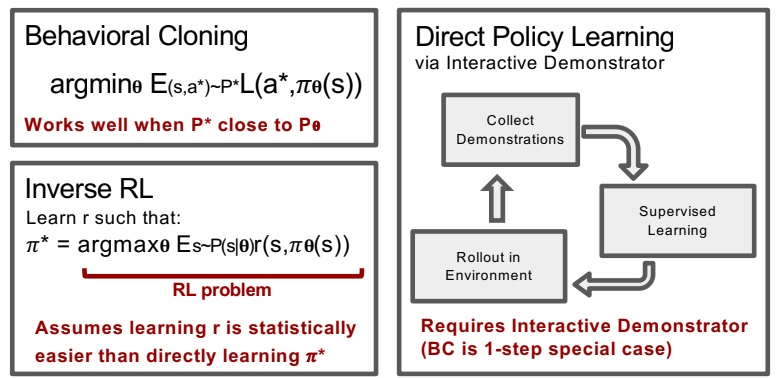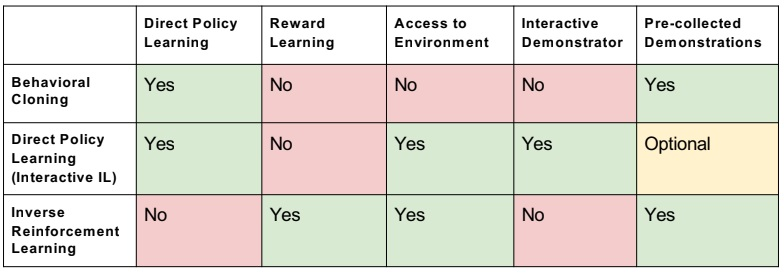### Dagger: Dataset Aggregation: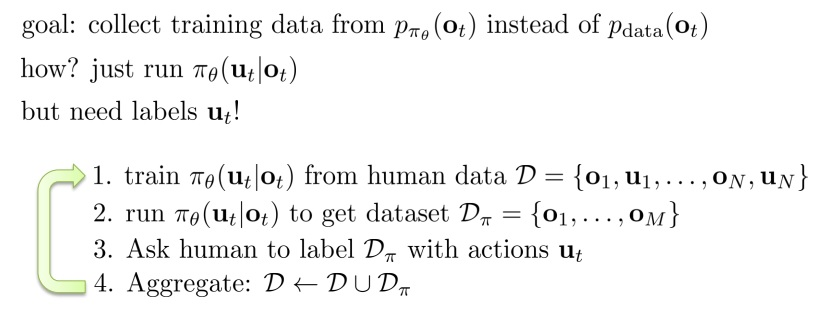### PLATO: Policy Learning with Adaptive Trajectory Optimization: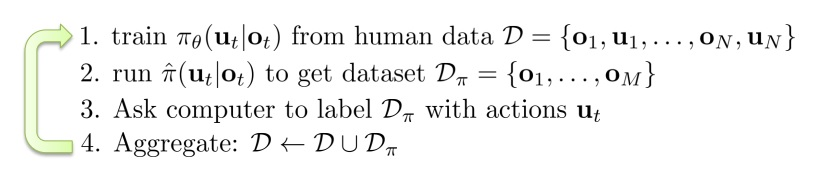## Meta-Learning:

• Meta-learning = learning to learn
• Supervised meta-learning = supervised learning with datapoints that are entire datasets
• If we can meta-learn a faster reinforcement learner, we can learn new tasks efficiently!
• What can a meta-learned learner do differently? 1.Explore more intelligently, 2.Avoid trying actions that are know to be useless, 3.Acquire the right features more quickly.
• The promise of meta-learning: use past experience to simply acquire a much more efficient deep RL algorithm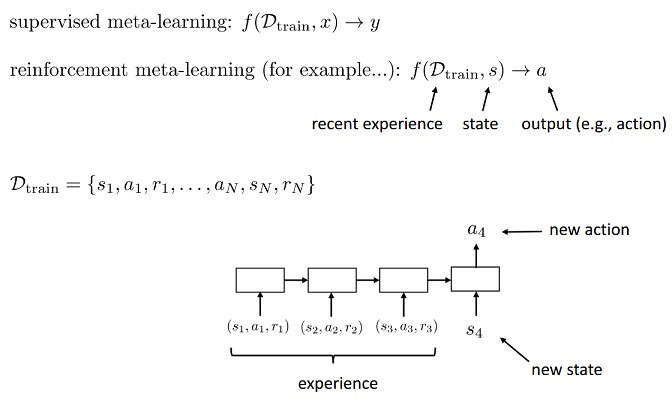## Neural Style Transfer Implementation

Neural Style Transfer is a method of creating artistic style images using Deep Neural Networks (Convolutional Neural Networks). This algorithm was created by Gatys et al. (2015) (https://arxiv.org/abs/1508.06576). Code is adapted from Andrew Ng’s Course ‘Convolutional Neural Networks”.

## Results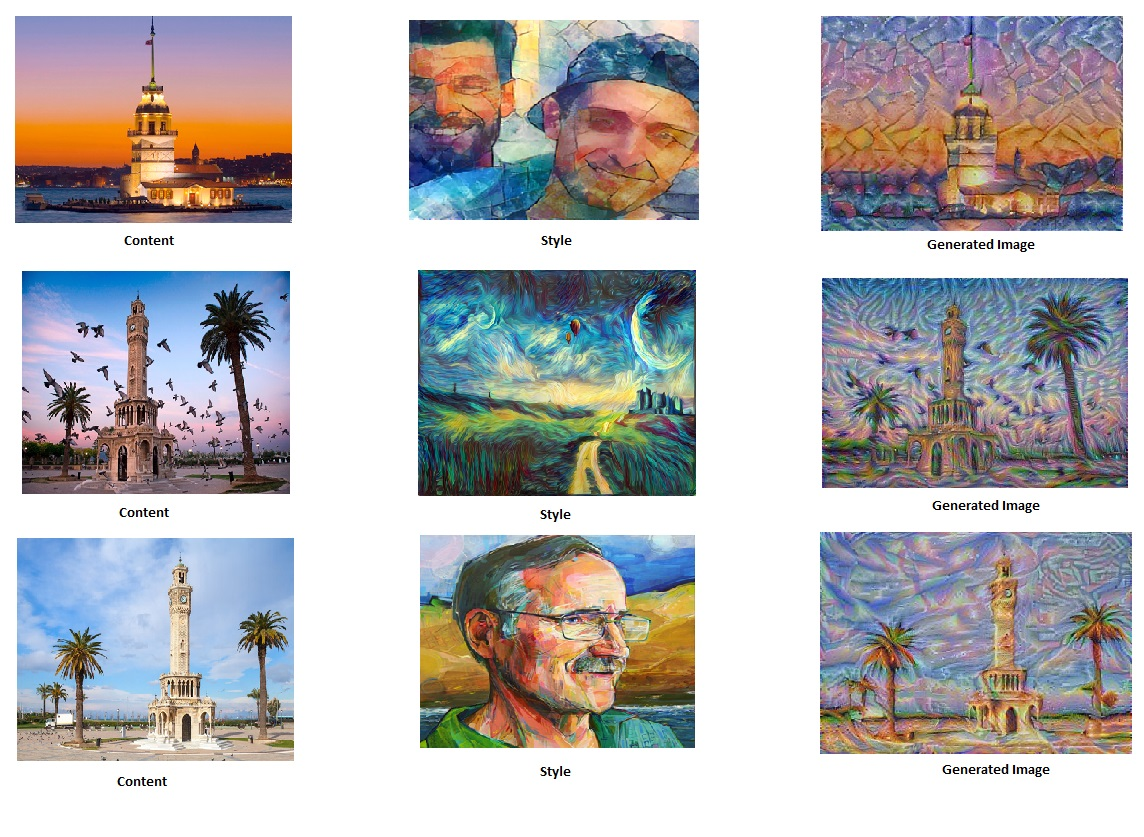## Transfer Learning

“Following the original NST paper (https://arxiv.org/abs/1508.06576), we will use the VGG network. Specifically, we’ll use VGG-19, a 19-layer version of the VGG network. This model has already been trained on the very large ImageNet database, and thus has learned to recognize a variety of low level features (at the earlier layers) and high level features (at the deeper layers)”

## Neural Style Transfer Algorithm

• Calculate the content cost function
• Calculate the style cost function
• Put it together to get $J(G) = \alpha J_{content}(C,G) + \beta J_{style}(S,G)$.
• Create an Interactive Session (tensorflow)
• Load the content image
• Load the style image
• Randomly initialize the image to be generated
• Load the VGG16 model
• Build the TensorFlow graph:
• Run the content image through the VGG16 model and compute the content cost
• Run the style image through the VGG16 model and compute the style cost
• Compute the total cost
• Define the optimizer and the learning rate
• Initialize the TensorFlow graph and run it for a large number of iterations, updating the generated image at every step.

## What is Transfer Learning? Transfer Learning With Keras (ResNet50)

“Transfer learning is a machine learning technique where a model trained on one task is re-purposed on a second related task. Transfer learning is an optimization that allows rapid progress or improved performance when modeling the second task.” If training and test sets are too small to implement ML application, transfer learning is very common technique that is used for avoiding overfitting in ML application. However there are two main points that are considering: inputs of pre-trained model and transferred model must be same and  transferred model size (e.g. 1000) is smaller than pre-trained one (e.g. 1000000).  Commonly, researchers use pre-trained models  in the frameworks. For instance, Keras library added pre-trained RESNET model with trained ImageNet datasets.

With following snippet, we can use Residual  Nets (ResNet50) from Keras Library for transfer learning.

def get_model():

input_tensor = Input(shape=(224, 224, 3))  # this assumes K.image_data_format() == 'channels_last'

# Create the base pre-trained model
base_model = ResNet50(input_tensor=input_tensor,weights='imagenet',include_top=False)

# Except last layer, other layers training is frozen with 'layer.trainable=false'
for layer in base_model.layers:
layer.trainable=False

# Pooling and new softmax layers added for new application
x = base_model.output
x = GlobalAveragePooling2D(data_format='channels_last')(x)
x = Dense(num_classes, activation='softmax')(x)

# Model is updated
updatedModel = Model(base_model.input, x)

return updatedModel

## Sentiment Analysis Using LSTM and GLoVe Word Embedding

Sentiment Analysis is an analysis of the sentence, text at the document that gives us the opinion of the sentence/text. In this project, it will be implemented a model which inputs a sentence and finds the most appropriate emoji to be used with this sentence. Code is adapted from Andrew Ng’s Course ‘Sequential Models’.

Github Code: https://github.com/omerbsezer/SentimentAnalysis

## Results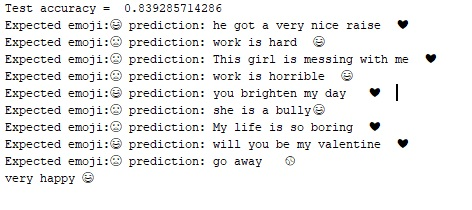## DataSet

We have a tiny dataset (X, Y) where:

• X contains 127 sentences (strings)
• Y contains a integer label between 0 and 4 corresponding to an emoji for each sentence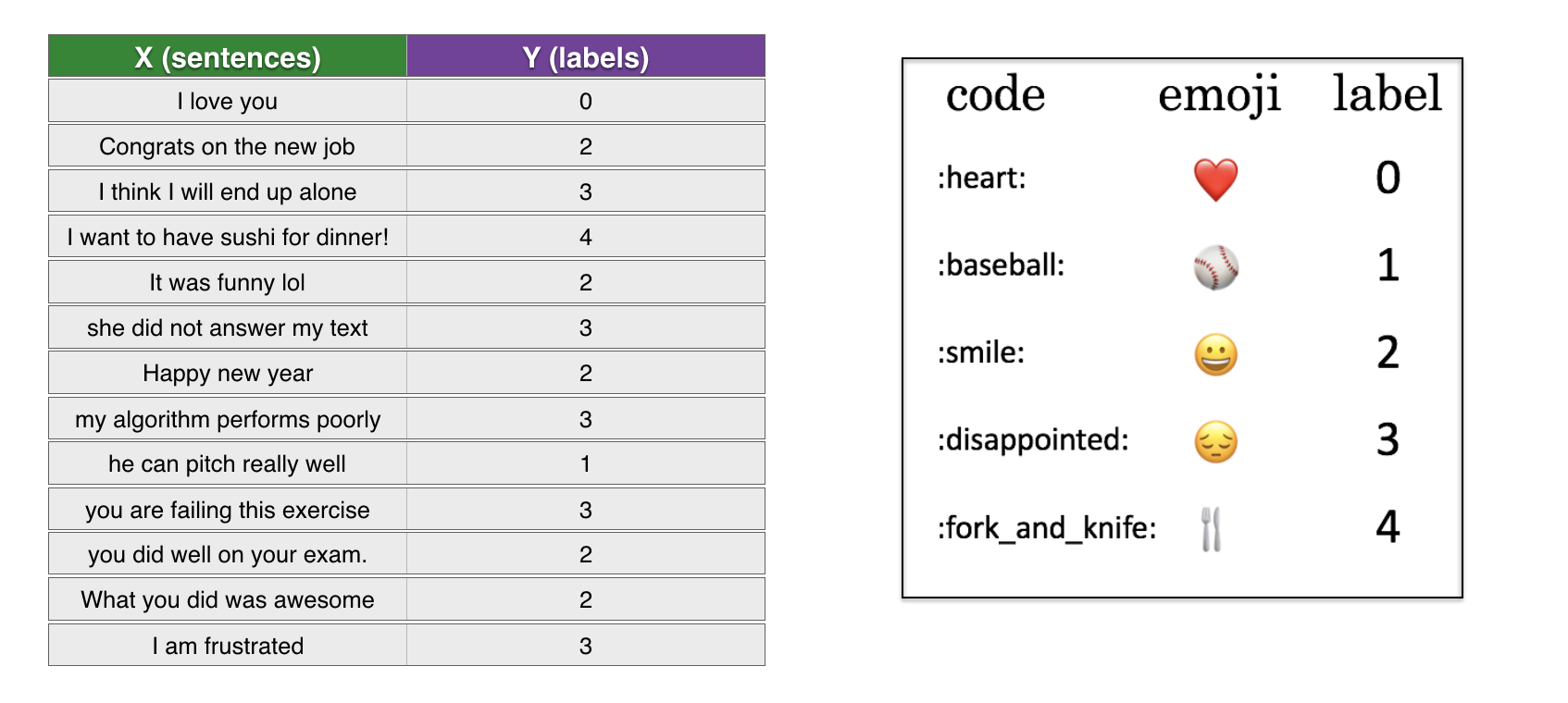## Embeddings

Glove 50 dimension, 40000 words of dictionary file is used for word embeddings. It should be downloaded from https://www.kaggle.com/watts2/glove6b50dtxt (file size = ~168MB))

• word_to_index: dictionary mapping from words to their indices in the vocabulary (400,001 words, with the valid indices ranging from 0 to 400,000)
• index_to_word: dictionary mapping from indices to their corresponding words in the vocabulary
• word_to_vec_map: dictionary mapping words to their GloVe vector representation.

## LSTM

LSTM structure is used for classification.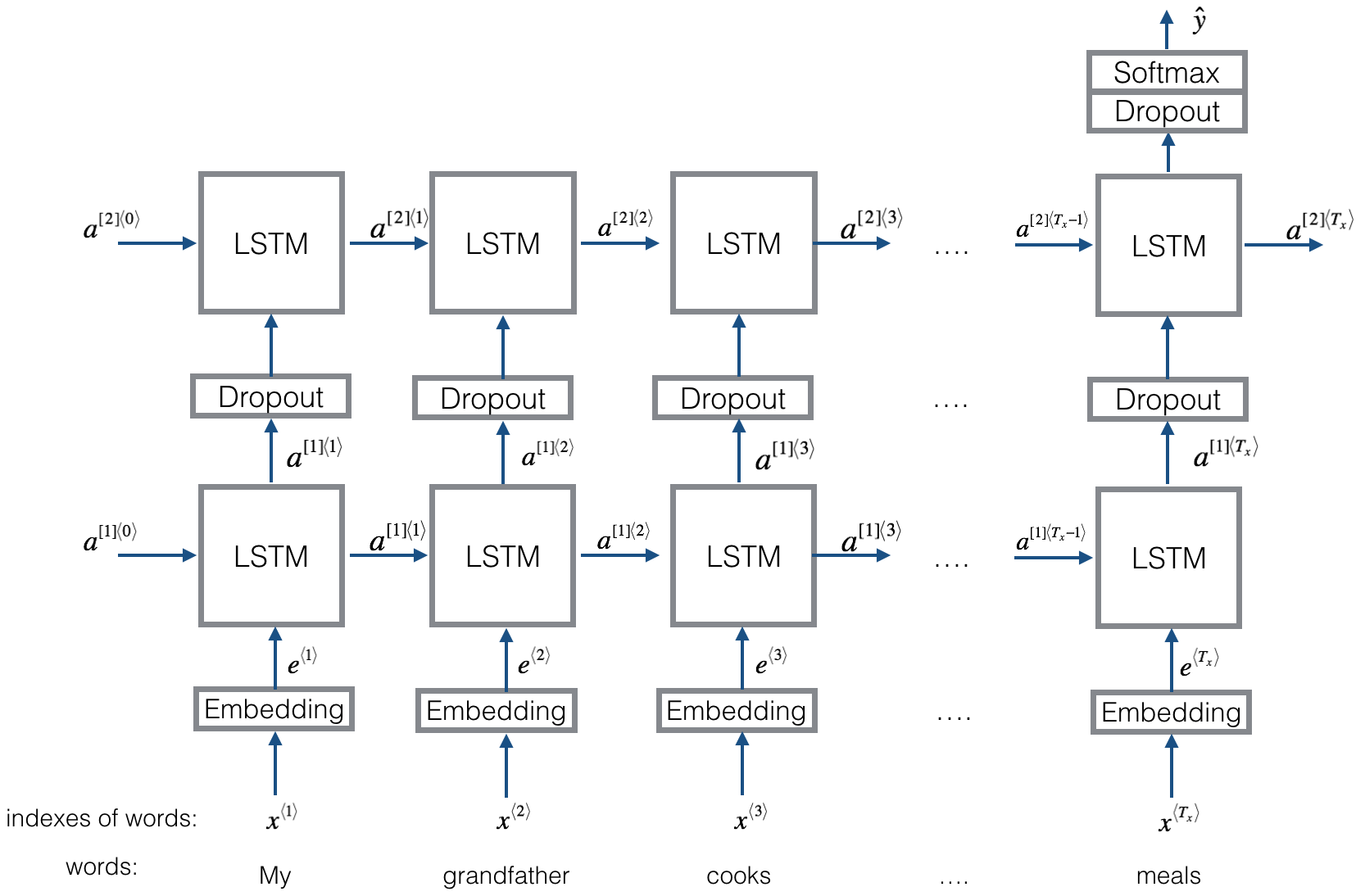Parameters: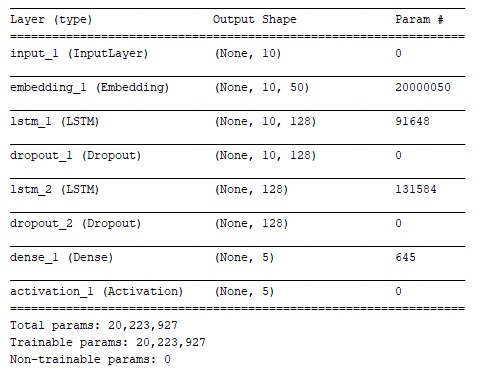## References

• Andrew Ng, Sequential Models Course, Deep Learning Specialization